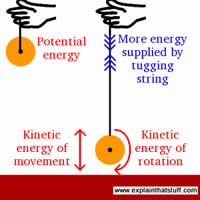# Difference between Kinetic Energy and Potential Energy

##### Key Difference: Kinetic energy is the energy of an object in motion. Potential energy is the energy that an object has when it is at rest.

Energy is available in many forms such as thermal energy, gravitational energy, nuclear energy, chemical energy, electric energy, elastic energy, electromagnetic radiation and rest energy. All of these forms of energy can be divided into two types: kinetic energy and potential energy. Energy cannot be created or destroyed and can only be transferred from one place to another. These two states of energy differ from each other in various ways, but they also work together in nature. Energy changes from potential to kinetic and then back to potential. Both the energies are measures in joules (J).Kinetic energy is the energy of an object in motion. Any object when it is travelling or moving has kinetic energy. The word ‘kinetic’ originates from Greek word, ‘kinesis’ which means “motion.” It is defined as the energy needed to accelerate a body of given mass from the state of rest to the state of motion. Kinetic energy is gained during acceleration and the object maintains the kinetic energy unless the speed changes and kinetic energy is lost when the object enters a state of rest again. In mechanics, the kinetic energy of a non-rotating object of mass ‘m’, which is travelling at speed ‘v’, is represented as ½ mv².

A system of bodies is considered to have internal kinetic energy due to the relative motion of the bodies around the system. Kinetic energy can also be transferred from one object to another through collision, such as in the case of billiards, where the cue ball transfers kinetic energy from itself to another ball. For example, our solar system is believed to have internal kinetic energy as the planets orbit around the Sun. Let’s understand it using various examples. A cyclist stationary on top of the hill starts freewheeling down the hill. The energy that created is kinetic energy. Now think of a waterfall, the flowing water also creates kinetic energy. This energy can also be harnessed and converted into other forms of energy such as electricity by using a watermill. Last example, a roller coaster when it goes up a hill uses kinetic energy and once it reaches the top, it rolls down the other side which is also kinetic energy.On the other hand, potential energy is the energy that an object has when it is at rest due to the position of the body. The term ‘potential energy’ was coined by 19th century Scottish engineer and physicist William Rankine. Potential energy is said to exist whenever an object which has a given mass has a position within a force field. It is force of an object that is still and not moving. Potential energy is also known as restoring energy of an object such as spring or gravitational force. Energy is converted into potential energy, when a moving object stops. In case of a spring, a stretched spring that is let go results in it going back to its original wound up position. This force is the result of potential energy, which is also known as restoring energy. Potential energy is represented as PE = mgh, where m is the mass, g is the gravitational acceleration of the earth and h is the height above the earth’s surface.

A more formal definition of potential energy is the difference of energy between the energy of an object in a given position and object’s energy at a reference point. There are various different types of potential energy such as elastic potential energy, gravitational potential energy, electric potential energy, nuclear potential energy, intermolecular potential energy and chemical potential energy. Examples of potential energy include the bike that used kinetic energy to rise to the top of the hill will stop at the hill before freewheeling down the other side. The pause at the top of the hill is potential energy which is then converted back to kinetic energy, now when the bike stops at the bottom of the hill the kinetic energy then converts back into potential energy. Another example is imagine a rubber ball, when it’s not moving and stationary it has potential energy, when a person throws the ball in the air, the energy converts to kinetic energy, when the balls falls back to the ground and stays on the ground its is converted back into potential energy.

In this sense, both potential energy and kinetic energy work together in almost everything.  Potential energy cannot exist without kinetic energy and vice-versa. Since energy cannot be destroyed or created, energy must exist in one or the other states, either potential or kinetic.

Image Courtesy: explainthatstuff.com, citruscollege.edu